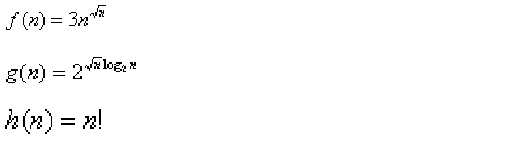# Data Structures and Algorithms | Set 8

• Difficulty Level : Hard
• Last Updated : 27 Mar, 2017

Following questions have been asked in GATE CS exam.

1. Consider the following functionsWhich of the following is true? (GATE CS 2000)

(a) h(n) is 0(f(n))
(b) h(n) is 0(g(n))
(c) g(n) is not 0(f(n))
(d) f(n) is 0(g(n))

g(n) = 2√n Log n = n√n

f(n) and g(n) are of same asymptotic order and following statements are true.
f(n) = O(g(n))
g(n) = O(f(n)).

(a) and (b) are false because n! is of asymptotically higher order than n√n.

2. Let G be an undirected connected graph with distinct edge weight. Let emax be the edge with maximum weight and emin the edge with minimum weight. Which of the following statements is false? (GATE CS 2000)

(a) Every minimum spanning tree of G must contain emin
(b) If emax is in a minimum spanning tree, then its removal must disconnect G
(c) No minimum spanning tree contains emax
(d) G has a unique minimum spanning tree

(a) and (b) are always true.
(c) is false because (b) is true.
(d) is true because all edge weights are distinct for G.

3. Let G be an undirected graph. Consider a depth-first traversal of G, and let T be the resulting depth-first search tree. Let u be a vertex in G and let v be the first new (unvisited) vertex visited after visiting u in the traversal. Which of the following statements is always true? (GATE CS 2000)

(a) {u,v} must be an edge in G, and u is a descendant of v in T
(b) {u,v} must be an edge in G, and v is a descendant of u in T
(c) If {u,v} is not an edge in G then u is a leaf in T
(d) If {u,v} is not an edge in G then u and v must have the same parent in T

See http://geeksquiz.com/data-structures-graph-question-20/ for explanation.

4. Consider an undirected unweighted graph G. Let a breadth-first traversal of G be done starting from a node r. Let d(r, u) and d(r, v) be the lengths of the shortest paths from r to u and v respectively, in G. lf u is visited before v during the breadth-first traversal, which of the following statements is correct? (GATE CS 2001)

a) d(r, u) < d (r, v) b) d(r, u) > d(r, v)
c) d(r, u) <= d (r, v) d) None of the above Answer (c) d(r, u) and d(r, v) will be equal when u and v are at same level, otherwise d(r, u) will be less than d(r, v)
5. How many undirected graphs (not necessarily connected) can be constructed out of a given set V= {V 1, V 2,…V n} of n vertices ? (GATE CS 2001)

a) n(n-l)/2
b) 2^n
c) n!
d) 2^(n(n-1)/2)